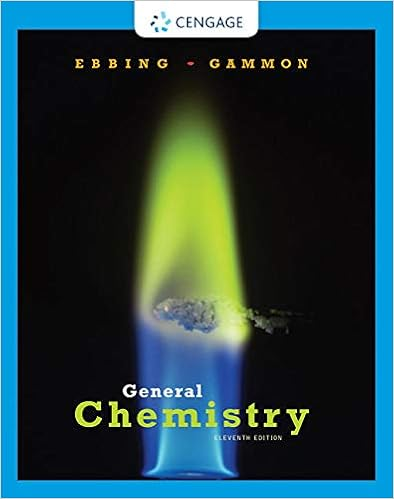# Consider the reaction 2so 2 g o 2 g 2so 3 g if a

• Test Prep
• 18
• 100% (15) 15 out of 15 people found this document helpful

This preview shows page 16 - 18 out of 18 pages.

##### We have textbook solutions for you!
The document you are viewing contains questions related to this textbook.The document you are viewing contains questions related to this textbook.
Chapter 20 / Exercise 20.102
General Chemistry
Ebbing/GammonExpert Verified
28.Consider the reaction 2SO2(g) + O2(g) 2SO3If a flask initially has 2.0 atm of SO2(g) and 2.0 atm of O2(g), what will the pressure beonce the reaction proceeds to completion, considering allgases present then? Assume constant volume and temperature.(g)A.1.0 atmB. 2.0 atmC. 3.5 atmD. 3.0 atmE. 4.0 atmAnswer: D. 3.0 atmPV = nRT Since V and T are constant, the moles of gas, n, is directly proportional to the pressure. Since all of the substances in the reaction are gases, we can use pressure instead of moles for the molar ratio calculations, instead of converting pressures to moles.We have to find the limiting reactant.Pressure of SO3produced by 2.0 atm of SO2:32322 mol SO2.0 atm SO= 2.0 atm SO2 atm SOPressure of SO3produced by 2.0 atm of O2:32322 mol SO2.0 atm O= 4.0 atm SO1 atm OSince less SO3is produced by the SO2, SO2is the limiting reactant; all of the SO2is consumed and 2.0 atm of O2is produced.O2is the excess reactant; find the amount left unreacted:
##### We have textbook solutions for you!
The document you are viewing contains questions related to this textbook.The document you are viewing contains questions related to this textbook.
Chapter 20 / Exercise 20.102
General Chemistry
Ebbing/GammonExpert Verified
22221 mol O2.0 atm SO= 1.0 atm O2 atm SOAt the end of the reaction that is 0 atm of SO2, 2.0 atm of SO3, and 1.0 atm of O2, for a total pressure of 3.0 atm.
29.Consider two 1-L samples of gas: one is H2and the other is O2. Both are at 1 atm and25°C. Twoof the following are the same for both samples. Select bothcorrect answers. (Yes, you fill in twobubbles on your scantron for THIS question. Both must be correct to receive credit for the question.)
•••